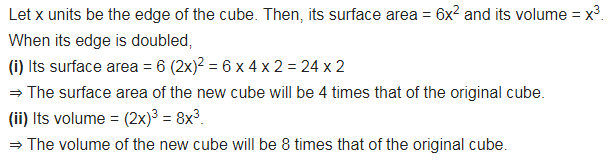# If each edge of a cube is doubled, (i) how many times will its surface area increase?

If each edge of a cube is doubled,
(i) how many times will its surface area increase?
(ii) how many times will its volume increase?